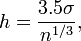## Saturday, May 01, 2010

### Matlab Programs

Last week I finally published two matlab programs that you might find useful. In this post I will describe the programs, why I made them and how you can use them.

In my opinion, Matlab's built in Error-bar function could be a lot better. By default it plots a spindly blue wisp of an error bar connected to its little friends. People that I know mostly use errorbars for bar plots, where this kind of graphic looks ridiculous. It is possible to modify parameters and setting in order to get matlabs errorbars to look the way you want, but as a result of an hour of confusion and a function call that runs off the page.
Also, it is extremely difficult to plot errorbars onto a group bar plot. My function, errorb or "create healthy looking error bars" takes the same input as Matlab's function and plots nice error bars as a default. It can also plot in color, just the tops, with varying linewidths and different widths of the little hats on top. It can also plot the bars horizontally.
Here is a screenshot from a function call:
The function is free and you can download it here: error bars.

The next function I tackled is the histogram function. In case you don't know what a histogram is I'm going to start with the screenshot:

If you call Matlab's histogram function, by default it breaks the range of your data into 10 bins and bar-plots it. In case you want more bins you can specify the number, but if you have a few outliers (points far from the main distribution) then its hard to capture it on one plot. Even then you can still change settings to make things reasonable but if you have to do it "by hand" for each plot you make. Things become even more confusing if you need to compare different graphs. This function will make all these tasks much easier as well as doing some fancy things you might not have asked for like output text that you might want to use for a figure caption.

Summary of what function does:
1. Automatically sets the number and range of the bins to be appropriate for the data.
2. Compares multiple sets of data elegantly on one or more plots, with legend or titles. It also graphs the mean and standard deviations. It can also plot the median and mode of each plot.
3. Outputs text with the useful statistics for each distribution.
4. Allows for changing many more parameters
There are really a lot of features that this function has, and you can see them all in the help file in the header of the function. It will also show you how to use it. On this page I'm just going to say what the function can do in an attempt to convince you to use it.

The key advantage of this function is that it allows you to plot multiple sets of data all at once, and for easy comparison. The default behaviour plots the discrete probability density of each one together by normalizing the area under the histogram to one. Also, to make things comparable when there are different bin sizes, the bin sizes are automatically set to be multiples of each other. In case you don't like different bin sizes, you can also choose to have them plotted with identical bins. In case you don't like them all on one plot then you can also have them plotted above each other on separate plots, but with the same axis bounds so they are stil comparable. In this case the legend will be replaced with subplot titles. But then your figure may become squished once you plot a few graphs. If you think this may be a problem you can set it to automatically expand the figure size if you are plotting a lot of data.

Note that the mean and standard deveation of the
distribution are by default plotted above the graph, but you can also turn it off. Also, the median and or the mode can be added to the graph with a stem plot.

The 'optimal' bin size that is chosen is actually a theoretical measure from "Scott's choice"where 'h' is the bin width, sigma is the standard deviation and 'n' is the number of data points in the set. The axis bounds are chosen by my own simple algorithm of setting it to be 4 times the standard deviation away from the mean (or the minimum of the data set). You can change this number from 4 to any you like, or set hard axis bounds.

The function returns a string with the plotname, mean, standard deviation, and the number of points that may fall outside the range plotted. You can also ask it to return a lot more text, including median, mode, number of total points and standard error.

The function can also return the number of items in each bin,
and the locations of the left edges of each bin.
The function is free and you can download it here: Plot and compare nice histograms by default

Lastly I have to thank Eli, Avi and the AP-Lab for all their help in designing these functions.

And on a side note: I now have an official "Authors" page on the Mathworks website, where I am ranked 978 with 120 downloads in just under a week. Lets hope this goes up!

1.2.3.